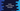# Kotlin take method explanation with different examples# Kotlin take() method :

take() method is defined in Kotlin standard library. This method can be used with an array or iterable. In this tutorial, we will learn how to use take _method in _kotlin with example. The program will show you how take _works on _arrays with a different data type.

## take() method :

The take() method is defined under kotlin.collections of Kotlin standard library.

``````fun  Array.take(n: Int): List
fun ByteArray.take(n: Int): List
fun ShortArray.take(n: Int): List
fun IntArray.take(n: Int): List
fun LongArray.take(n: Int): List
fun FloatArray.take(n: Int): List
fun DoubleArray.take(n: Int): List
fun BooleanArray.take(n: Int): List
fun CharArray.take(n: Int): List
fun  Iterable.take(n: Int): List``````

As you can see that we can use take() method with array or with iterables. We will check how it works with different examples below.

It takes one integer n as parameter and returns a list.

## Example of take method with arrays :

``````import java.util.*

fun main(args: Array) {
val intArray = intArrayOf(1, 2, 3, 4, 5)
val charArray = charArrayOf('a', 'b', 'c', 'd', 'e')
val floatArray = floatArrayOf(1.0f, 2.0f, 3.0f)

println("First 3 values of \${Arrays.toString(intArray)} are \${intArray.take(3)}")
println("First 2 values of \${Arrays.toString(charArray)} are \${charArray.take(2)}")
println("First 5 values of \${java.util.Arrays.toString(floatArray)} are \${floatArray.take(5)}")
}``````

If you run this program, it will print the below output :

``````First 3 values of [1, 2, 3, 4, 5] are [1, 2, 3]
First 2 values of [a, b, c, d, e] are [a, b]
First 5 values of [1.0, 2.0, 3.0] are [1.0, 2.0, 3.0]``````
1. In this example, we have tried take() method with three different arrays.
2. The return value is a list containing the first_ n_ elements of each array, where n is the argument.
3. The last array has 3 elements, but we are passing 5 as the argument. So it returned a list containing all elements of the array.

## Example with Iterable :

Let’s try to implement the above program with a list and set :

``````fun main(args: Array) {
val list = listOf(1,2,3,4,5)
val set = setOf(1,2,2,4,5,6)

println("First 3 values of \$list are \${list.take(3)}")
println("First 7 values of \$set are \${set.take(7)}")
}``````

It will print :

``````First 3 values of [1, 2, 3, 4, 5] are [1, 2, 3]
First 7 values of [1, 2, 4, 5, 6] are [1, 2, 4, 5, 6]``````

So, the same rule is applied with list and set. The first variable is a list and the second is a set.

The above examples are available on Github